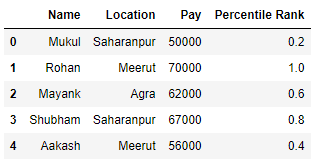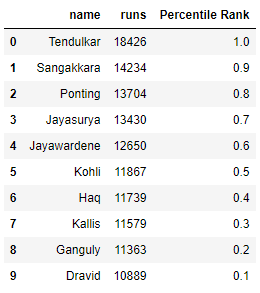Related Articles

# Percentile rank of a column in a Pandas DataFrame

• Last Updated : 17 Aug, 2020

Let us see how to find the percentile rank of a column in a Pandas DataFrame. We will use the `rank()` function with the argument `pct = True` to find the percentile rank.

Example 1 :

 `# import the module``import` `pandas as pd `` ` `# create a DataFrame ``data ``=` `{``'Name'``: [``'Mukul'``, ``'Rohan'``, ``'Mayank'``, ``                 ``'Shubham'``, ``'Aakash'``],``        ``'Location'` `: [``'Saharanpur'``, ``'Meerut'``, ``'Agra'``, ``                      ``'Saharanpur'``, ``'Meerut'``],``        ``'Pay'` `: [``50000``, ``70000``, ``62000``, ``67000``, ``56000``]} ``df ``=` `pd.DataFrame(data)  `` ` `# create a new column of percentile rank``df[``'Percentile Rank'``] ``=` `df.Pay.rank(pct ``=` `True``)`` ` `# displaying the percentile rank``display(df) `

Output :Example 2 :

 `# import the module``import` `pandas as pd `` ` `# create a DataFrame ``ODI_runs ``=` `{``'name'``: [``'Tendulkar'``, ``'Sangakkara'``, ``'Ponting'``, ``                      ``'Jayasurya'``, ``'Jayawardene'``, ``'Kohli'``, ``                      ``'Haq'``, ``'Kallis'``, ``'Ganguly'``, ``'Dravid'``], ``            ``'runs'``: [``18426``, ``14234``, ``13704``, ``13430``, ``12650``, ``                     ``11867``, ``11739``, ``11579``, ``11363``, ``10889``]} ``df ``=` `pd.DataFrame(ODI_runs)  `` ` `# create a new column of percentile rank``df[``'Percentile Rank'``] ``=` `df.runs.rank(pct ``=` `True``)`` ` `# displaying the percentile rank``display(df) `

Output :Attention geek! Strengthen your foundations with the Python Programming Foundation Course and learn the basics.

To begin with, your interview preparations Enhance your Data Structures concepts with the Python DS Course. And to begin with your Machine Learning Journey, join the Machine Learning – Basic Level Course

My Personal Notes arrow_drop_up